基于深度学习的单通道语音增强

传统的语音增强方法基于一些设定好的先验假设，但是这些先验假设存在一定的不合理之处。此外传统语音增强依赖于参数的设定，人工经验等。随着深度学习的发展，越来越多的人开始注意使用深度学习来解决语音增强问题。由于单通道使用场景较多，本文就以单通道语音增强为例。

目前基于深度神经网络单通道语音增强方法大致可以分为两类：

• 第一种基于映射的方法

基于映射的语音增强

基于映射的语音增强方法通过训练神经网络模型直接学习带噪语音和纯净语音之间的映射关系，有两种映射方案：

我们以频谱映射举例说明一下：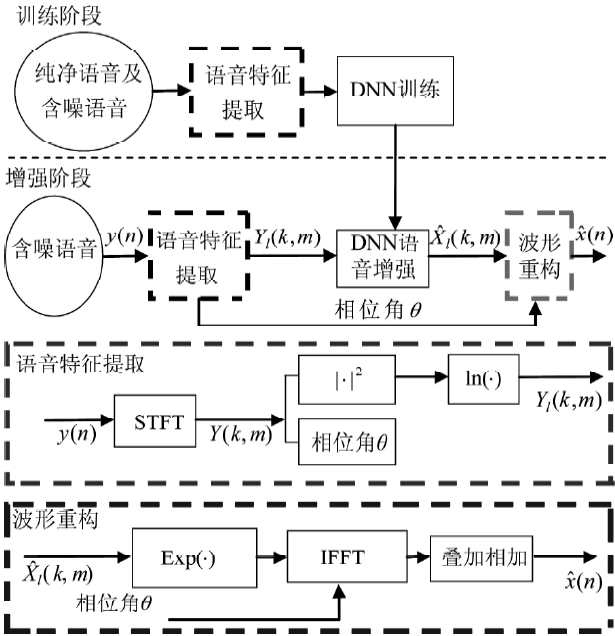训练阶段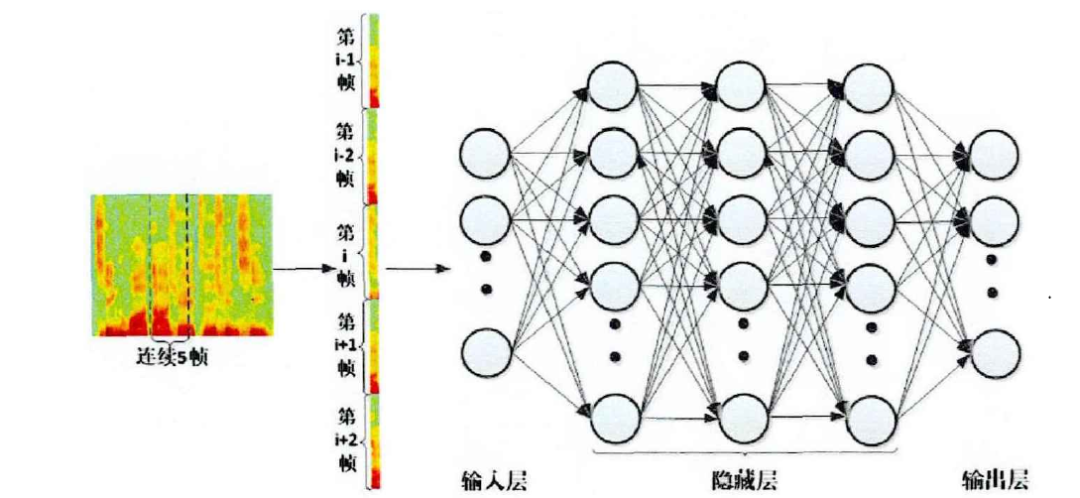• Label：数据的label为纯净语音信号的幅度谱，这里只需要一帧就够了。
• 损失函数：学习噪声幅度谱与纯净语音信号的幅度谱类似于一个回归问题，因此损失函数采用回归常用的损失函数，如均方误差（MSE）、均方根误差（RMSE）或平均绝对值误差（MAE）等....
• 最后一层的激活函数：由于是回归问题，最后一层采用线性激活函数
• 其他：输入的幅度谱进行归一化可以加速学习过程和更好的收敛。如果不采用幅度谱可以采用功率谱，要注意的是功率谱如果采用的单位是dB，需要对数据进行预处理，因为log的定义域不能为0，简单的方法就是在取对数前给等于0的功率谱加上一个非常小的数

增强阶段

• 输入：输入为噪声信号的幅度谱，这里同样需要扩帧。对输入数据进行处理可以在语音信号加上值为0的语音帧，或者舍弃首尾的几帧。如果训练过程对输入进行了归一化，那么这里同样需要进行归一化
• 输出：输入为估计的纯净语音幅度谱
• 重构波形：在计算输入信号幅度谱的时候需要保存每一帧的相位信息，然后用保存好的相位信息和模型输出的幅度谱重构语音波形，代码如下所示。
spectrum = magnitude * np.exp(1.0j * phase)

时频掩蔽

我们都知道语音信号可以通过时域波形或者频域的各种频谱表示，此外语谱图可以同时展示时域和频域的信息，因此被广泛应用，如下图所示。语谱图上的像素点就可以称为 时频单元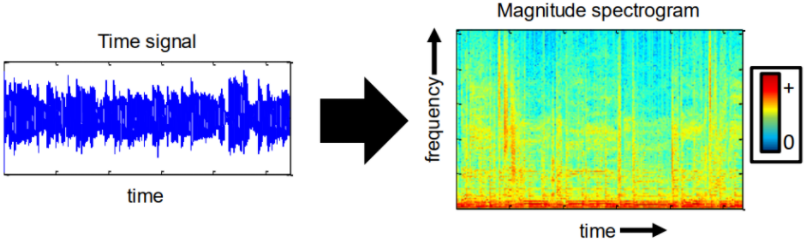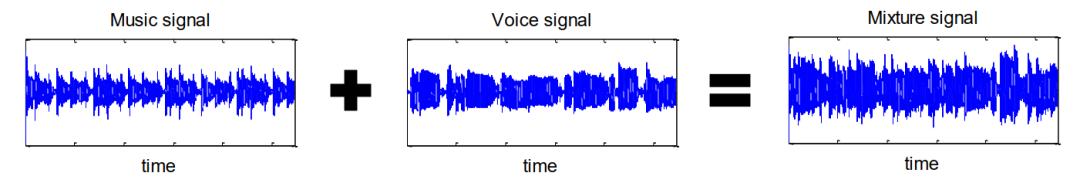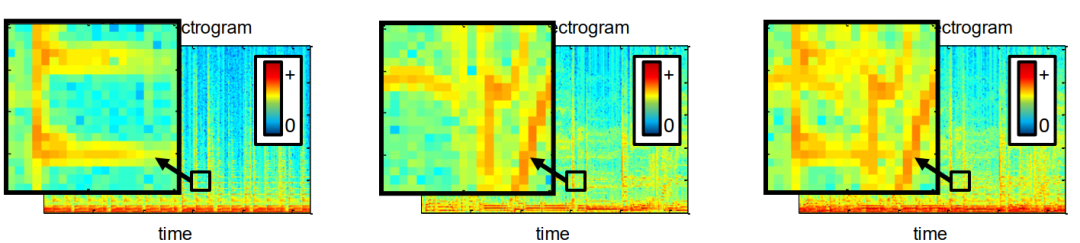1、我们假设信号能量稀疏的，即对于大多数时频区域它的能量为0，如下图所示，我们可以看到大多数区域的值，即频域能量为0。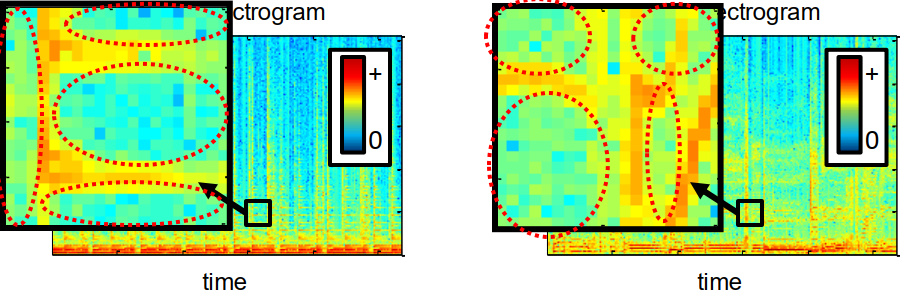2、我们假设信号能量不相交的，即它们的时频区域不重叠或者重叠较少，如下图所示，我们可以看到时频区域不为0的地方不重叠或者有较少部分的重叠。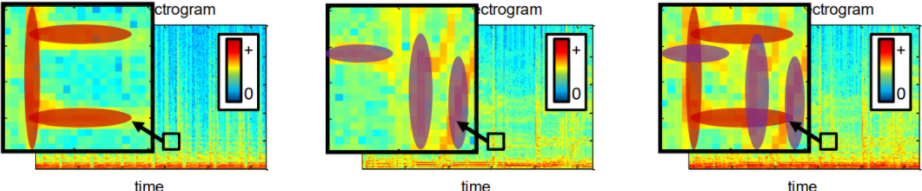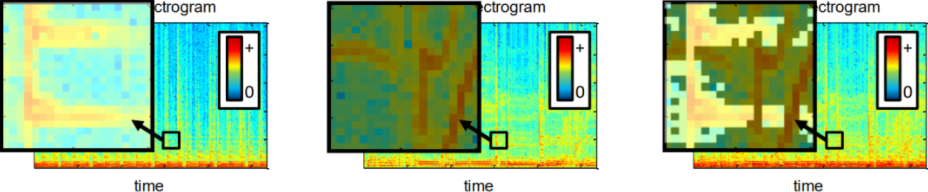我们通过0和1的二值掩码然后乘以混合信号的语谱图就可以得到我们想要喜好的语谱图了，如下图所示。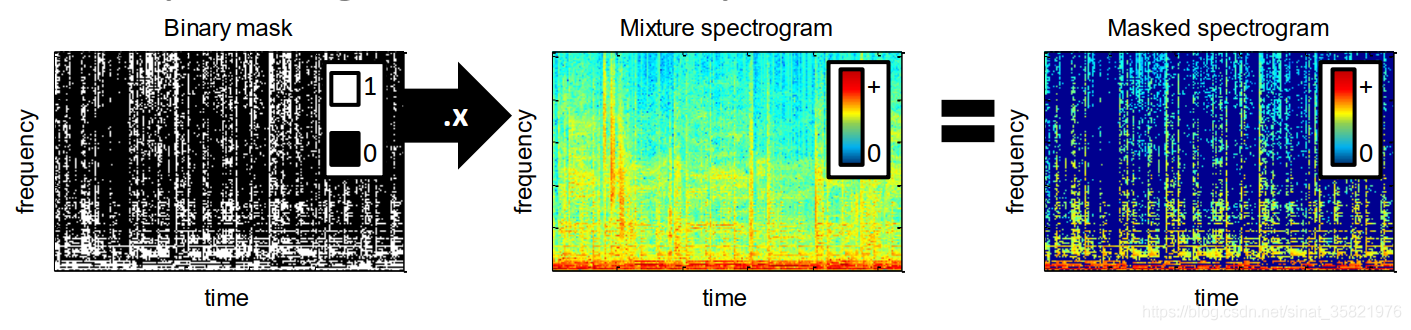神经模型一般直接预测时频掩蔽$M(t,f)$，之后再通过$M(t,f)$与混合语音$Y(t,f)$相乘得到预测的纯净语音$\hat{S}(t,f)=\hat{M}(t,f)\otimes Y(t,y)$，其中$\otimes$代表哈达玛乘积(Hadamard Product)。在语音增强研究的发展过程中，研究人员提出了一系列的时频掩蔽作为训练目标：

因此，IBM 的值由时频单元上的信噪比SNR(t,f)和设定的阈值比较之后决定：

$$公式1：I B M(t, f)=\left\{\begin{array}{l} 1,\quad if\quad |S(t,f)|^2-|N(t,f)|^2>\theta \\ 0,\quad \text { otherwise} \end{array}\right.$$

• 优点：IBM 作为二值目标，只需要使用简单的二分类模型进行预测，并且可以有效地提高语音的可懂度。
• 缺点：IBM 只有 0 和 1 两种取值，对带噪语音的处理过于粗暴，处理过程中引入了较大的噪声，无法有效地改善语音质量。

def IBM(clean_S, noise_S):
:param clean_S: 纯净语音 STFT
:param noise_S: 噪声 STFT
:return: 纯净语音的理想二值掩膜 IBM
"""def IBM_SNR(clean_speech, noise_speech):
Erdogan, Hakan, et al. "Phase-sensitive and recognition-boosted speech separation using deep recurrent neural networks." ICASSP, 2015.
:param clean_speech: 纯净语音 STFT
:param noise_speech: 带噪语音 STFT
:return: 纯净语音的理想二值掩膜 IBM
"""
_eps = np.finfo(np.float).eps  # 避免除以0
theta = 0.5  # a majority vote
alpha = 1  # ratio of magnitudes
mask = np.divide(np.abs(clean_speech) ** alpha, (_eps + np.abs(noise_speech) ** alpha))def IBM_SNR(clean_speech, noise_speech,delta_size):
Erdogan, Hakan, et al. "Phase-sensitive and recognition-boosted speech separation using deep recurrent neural networks." ICASSP, 2015.
:param clean_speech: 纯净语音 STFT
:param noise_speech: 带噪语音 STFT
:return: 纯净语音的理想二值掩膜 IBM
"""
_eps = np.finfo(np.float).eps  # 避免除以0
local_snr = 0
ibm  = np.where(10. * np.log10(np.abs(clean_speech) ** 2 / np.abs(noise_speech) ** 2) >= local_snr, 1., 0.)

if delta_size > 0:
ibm = ibm[:, delta_size: -delta_size]
return ibm
View Code

在这个假设下带噪语音的能量可以表示为：

$$公式2：|\boldsymbol{Y}(t, f)|^{2}=|\boldsymbol{S}(t, f)+\boldsymbol{N}(t, f)|^{2}=|\boldsymbol{S}(t, f)|^{2}+|\boldsymbol{N}(t, f)|^{2}$$

$$公式3：I R M(t, f)=\left(\frac{|S(t, f)|^{2}}{|Y(t, f)|^{2}}\right)^{\beta} =\left(\frac{|S(t, f)|^{2}}{|S(t, f)|^{2}+|N(t, f)|^{2}}\right)^{\beta}$$

• 优点：IRM 是分布在 0 到 1 的连续值，因此 IRM 是对目标语音更加准确的刻画，这使得 IRM 可以有效地同时提升语音的质量和可懂度。
• 缺点：使用未处理的相位信息进行语音重构（相位对于感知质量也很重要）
def IRM(clean_S, noise_S, beta=0.5):
:param clean_S: 纯净语音 STFT
:param noise_S: 噪音 STFT
"""
_eps = np.finfo(np.float).eps  # 防止分母出现0
mask = np.abs(clean_S) ** 2 / np.abs(clean_S) ** 2 + np.abs(noise_S) ** 2 + _eps
if beta:

$$公式4：\operatorname{IAM}(t, f)=\frac{|S(t, f)|}{|Y(t, f)|}$$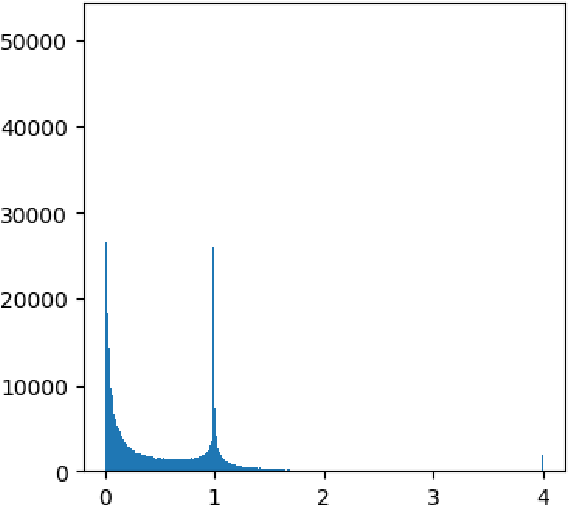def IAM(clean_S, noisy_S, clip=True):
:param clean_S: 纯净语音 STFT
:param noisy_S: 带噪语音 STFT
"""
_eps = np.finfo(np.float).eps  # 避免除以0
mask = np.abs(clean_S) / (np.abs(noisy_S) + _eps)
if clip:

PSM在形式上是 IAM 乘上纯净语音和带噪语音之间的余弦相似度

$$公式5：P S M(t, f)=\frac{|S(t, f)|}{|Y(t, f)|} \cos \left(\theta^{S}-\theta^{Y}\right)$$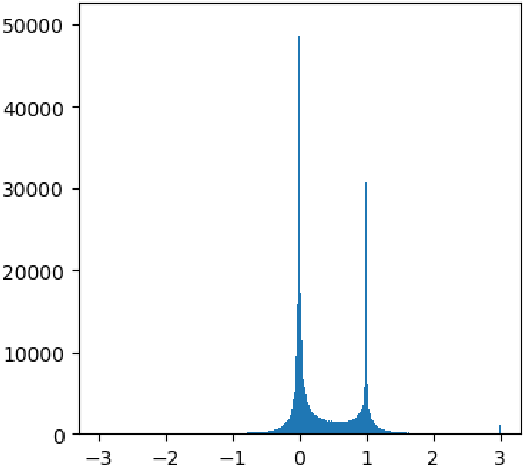PSM数值分布直方图

• 优点：纯净语音相位和带噪语音相位的差异，加入相位信息之后，PSM方法可以获得更高的SNR，因而降噪效果比IAM更好。
def PSM(clean_S, noisy_S):
:param clean_S: 纯净语音 STFT
:param noisy_S: 带噪语音 STFT
"""
_eps = np.finfo(np.float).eps  # 防止分母出现0

mask = (np.abs(clean_S) / (np.abs(noisy_S) + _eps)) * np.cos(np.angle(clean_S) - np.angle(noisy_S))
# Theta = np.clip(np.cos(np.angle(clean_S)-np.angle(noisy_S)), a_min=0., a_max=1.)
# mask = np.divide(np.abs(clean_S), _eps + np.abs(noisy_S)) * Theta

$$S(t,f)=M(t,f)*Y(t,f)$$

$*$是复数乘法，其中

$$\left\{ \begin{array}{l}Y = {Y_r} + i{Y_i}\\M = {M_r} + i{M_i}\\S = {S_r} + i{S_i}\end{array} \right.==>{S_r} + i{S_i} = ({M_r} + i{M_i})*({Y_r} + i{Y_i}) = ({M_r}{Y_r} - {M_i}{Y_i}) + i({M_r}{Y_i} + {M_i}{Y_r})$$

$$M_{cIRM} = {M_r} + i{M_i} = {\frac{{{Y_r}{S_r} + {Y_i}{S_i}}}{{Y_r^2 + Y_i^2}}} + i\frac{{{Y_r}{S_i} - {Y_i}{S_r}}}{{Y_r^2 + Y_i^2}}$$

• 优点：cIRM能够同时增强嘈杂语音的幅度和相位响应，cIRM是加性噪声假设下的最优掩蔽，可以从带噪语音中完美重构纯净语音信号
def cIRM(clean_S, noisy_S):
"""使用复理想比率掩码将语音从源信号的短时傅里叶变换和混合源信号的短时傅里叶变换中分离出来
:param clean_S:纯净语音 STFT
:param noisy_S:带噪语音 STFT
"""
clean_real = np.real(clean_S)
clean_imag = np.imag(clean_S)
noisy_real = np.real(noisy_S)
noisy_imag = np.imag(noisy_S)

cIRM_r = (noisy_real * clean_real + noisy_imag * clean_imag) / (noisy_real ** 2 + noisy_imag ** 2)
cIRM_i = (noisy_real * clean_imag - noisy_imag * clean_real) / (noisy_real ** 2 + noisy_imag ** 2)

mask = cIRM_r + cIRM_i * 1j

Liang S, Liu W, Jiang W, et al. The optimal ratio time-frequency mask for speech separation in terms of the signal-to-noise ratio[J]. The Journal of the Acoustical Society of America, 2013, 134(5): EL452-EL458.

ORM的定义是通过最小化纯净语音和估计目标语音的均方误差(MSE)来导出的

$$公式1：ORM(t, f)=\frac{|S(t, f)|^{2}+\mathcal{R}\left(S(t, f) N^{*}(t, f)\right)}{|S(t, f)|^{2}+|N(t, f)|^{2}+2 \mathcal{R}\left(S(t, f) N^{*}(t, f)\right)}$$

可以看出，ORM与IRM非常相似，不同之处在于相干部分$\mathcal{R}\left(S(t, f) N^{*}(t, f)\right)$，在IRM中假定其为0。事实上，这个假设太过强烈了。图2显示了带噪语音中纯净语音和噪声之间的频谱一致性。我们可以看到语音和噪声是高度相关的。ORM在中被证明在语音分离方面获得了更好的性能。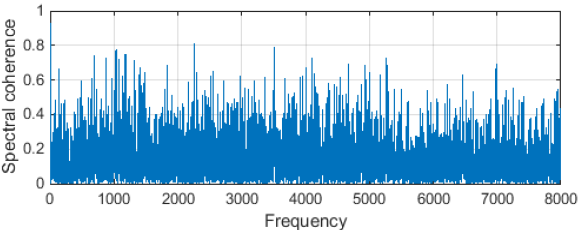ORM 在$(-\infty,+\infty)$的范围内变化，这是不容易估计的。 因此，我们用双曲正切来限制 ORM 的值

$$\mathrm{ORM}(t, f)=K \frac{1-e^{-c \gamma(t, f)}}{1+e^{-c \gamma(t, f)}}$$

def mask_ORM(clean_S, noise_S, K=10, C=0.1, compress=False):
"""
:param clean_S: 纯净语音的STFT
:param noise_S: 噪声的STFT
:param compress:是否限制
:return:
"""

mag_clean = np.abs(clean_S)
mag_noise = np.abs(noise_S)
coherent_part = np.real(clean_S * np.conj(noise_S))  # 相干部分
mask = (mag_clean ** 2 + coherent_part) / (mag_clean ** 2 + mag_noise ** 2 + 2 * coherent_part)
if compress:

总结

语音增强中的大部分掩蔽类方法，都可以看成在特定的假设条件下cIRM 的近似。如果将 cIRM 在直角坐标系下分解，cIRM 在实数轴上的投影就是 PSM。如果再将 cIRM在极坐标系下分解，cIRM 的模值就是 IAM。而 IRM 又是 IAM 在噪声语音不相关假设下的简化形式，IBM 则可以认为是 IRM 的二值版本。

上述排名并不代表所有模型和领域，像IRM、IBM等掩蔽方法，进行了某些特定的假设，所以都会在一定程度上造成性能损失。虽然 ORM 是最优掩蔽，但是使用其他简化的掩蔽方法可以降低预测的难度。这也是早期的语音增强研究选择使用 IBM 或者是 IRM 等简单掩蔽目标的原因。在模型容量有限的情况下，cIRM 经常并不是最好的选择，选择和模型建模能力匹配的目标才能获得最优的增强性能。

题外话

但是，这里存在一个问题，我们无法从语谱图中还原语音信号。为了解决这一问题，我们首先还原所有的频率分量，即对二值掩码做个镜像后拼接。假设我们计算语谱图时使用的是512点SFTF，我们一般去前257点进行分析和处理，在这里我们将前257点的后255做镜像，然后拼接在一起得到512点频率分量，如下图所示。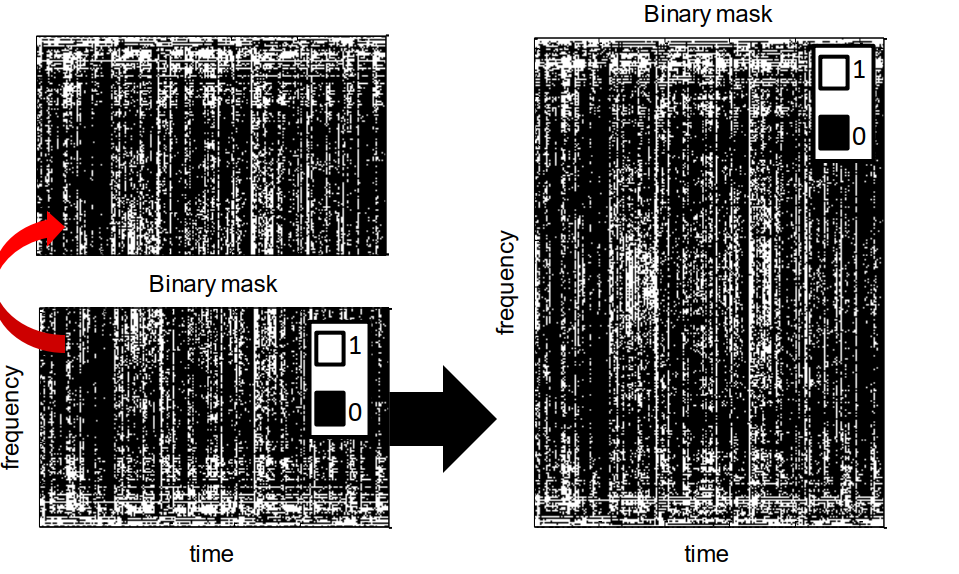• Label：数据的label为根据信噪比计算的IBM或者IRM，这里只需要一帧就够了
• 损失函数：IBM的损失函数可以用交叉熵，IRM的损失函数还是用均方差
• 最后一层的激活函数：IBM只有0和1两个值，IRM范围为[0,1]，因此采用sigmoid激活函数就可以了

Demo效果以及代码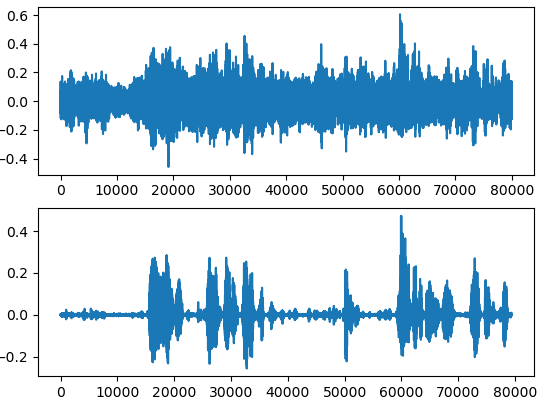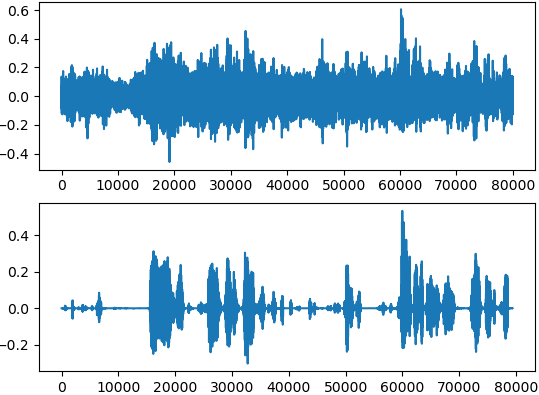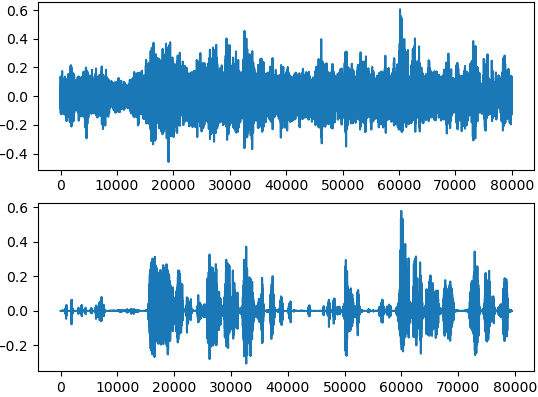"""
@FileName: IBM.py
@Description: Implement IBM
@Author: Ryuk
@CreateDate: 2020/05/08
@LastEditTime: 2020/05/08
@Version: v0.1
"""import numpy as np
import librosa
from sklearn.preprocessing import StandardScaler
from keras.layers import *
from keras.models import Sequential
​
​
def generateDataset():
​
win_length = 256
hop_length = 128
nfft = 512
​
mix_spectrum = librosa.stft(mix, win_length=win_length, hop_length=hop_length, n_fft=nfft)
clean_spectrum = librosa.stft(clean, win_length=win_length, hop_length=hop_length, n_fft=nfft)
​
mix_mag = np.abs(mix_spectrum).T
clean_mag = np.abs(clean_spectrum).T
​
​
frame_num = mix_mag.shape - 4
feature = np.zeros([frame_num, 257*5])
k = 0
for i in range(frame_num - 4):
frame = mix_mag[k:k+5]
feature[i] = np.reshape(frame, 257*5)
k += 1
​
snr = np.divide(clean_mag, mix_mag)
​
​
ss = StandardScaler()
feature = ss.fit_transform(feature)
return feature, label
​
​
def getModel():
model = Sequential()
​
​
​
​
return model
​
def train(feature, label, model):
loss='mse',
metrics=['mse'])
model.fit(feature, label, batch_size=128, epochs=20, validation_split=0.1)
model.save("./model.h5")
​
def main():
feature, label = generateDataset()
model = getModel()
train(feature, label, model)
​
​
if __name__ == "__main__":
main()

"""
@FileName: Inference.py
@Description: Implement Inference
@Author: Ryuk
@CreateDate: 2020/05/08
@LastEditTime: 2020/05/08
@Version: v0.1
"""import librosa
import numpy as np
from basic_functions import *
import matplotlib.pyplot as plt
from sklearn.preprocessing import StandardScaler
​
def show(data, s):
plt.figure(1)
ax1 = plt.subplot(2, 1, 1)
ax2 = plt.subplot(2, 1, 2)
plt.sca(ax1)
plt.plot(data)
plt.sca(ax2)
plt.plot(s)
plt.show()
​
​
​
win_length = 256
hop_length = 128
nfft = 512
​
spectrum = librosa.stft(data, win_length=win_length, hop_length=hop_length, n_fft=nfft)
magnitude = np.abs(spectrum).T
phase = np.angle(spectrum).T
​
frame_num = magnitude.shape - 4
feature = np.zeros([frame_num, 257 * 5])
k = 0
for i in range(frame_num - 4):
frame = magnitude[k:k + 5]
feature[i] = np.reshape(frame, 257 * 5)
k += 1
​
ss = StandardScaler()
feature = ss.fit_transform(feature)
​
fig = plt.figure()
plt.show()
plt.close(fig)
​
magnitude = magnitude[2:-2]
phase = phase[2:-2]
​
en_spectrum = en_magnitude.T * np.exp(1.0j * phase.T)
frame = librosa.istft(en_spectrum, win_length=win_length, hop_length=hop_length)
​
show(data, frame)
librosa.output.write_wav("./output.wav",frame, sr=8000)

参考

【论文】2017_Using Optimal Ratio Mask as Training Target for Supervised Speech Separation

【论文】2016_Complex ratio masking for monaural speech separation

【论文】2015_Phase-sensitive and recognition-boosted speech separation using deep recurrent neural networks

【论文】2020_李劲东_基于深度学习的单通道语音增强研究

【博客文章】DNN单通道语音增强(附Demo代码)